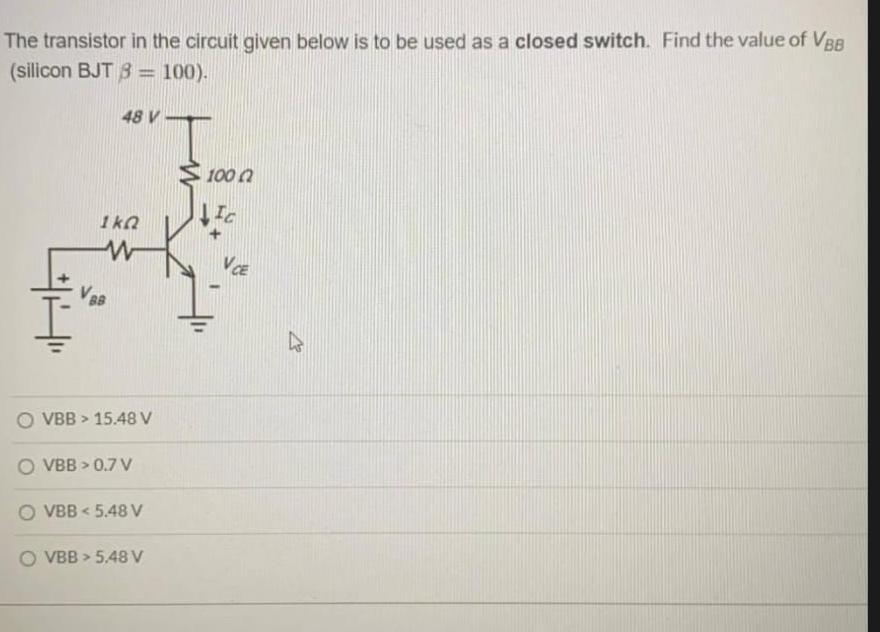Question:

# The transistor in the circuit given below is to be used as a closed switch. Find the value of VBB (silicon BJT 3 = 100). 48 V 10The transistor in the circuit given below is to be used as a closed switch. Find the value of VBB (silicon BJT 3 = 100). 48 V 1000 IC Ik W VCE V88 O VBB > 15.48 V O VBB > 0.7V VBB < 5.48 V VBB > 5.48 V